# Ways to apply an if condition in Pandas DataFrame

• Last Updated : 18 Aug, 2020

Generally on a Pandas DataFrame the if condition can be applied either column-wise, row-wise, or on an individual cell basis. The further document illustrates each of these with examples.

First of all we shall create the following DataFrame :

 `# importing pandas as pd``import` `pandas as pd`` ` `# create the DataFrame``df ``=` `pd.DataFrame({``    ``'Product'``: [``'Umbrella'``, ``'Matress'``, ``'Badminton'``, ``                ``'Shuttle'``, ``'Sofa'``, ``'Football'``],``    ``'MRP'``: [``1200``, ``1500``, ``1600``, ``352``, ``5000``, ``500``],``    ``'Discount'``: [``0``, ``10``, ``0``, ``10``, ``20``, ``40``]``})`` ` `# display the DataFrame``print``(df)`

Output :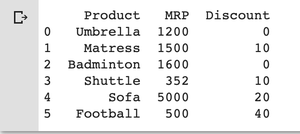Example 1 : if condition on column values (tuples) : The if condition can be applied on column values like when someone asks for all the items with the MRP <=2000 and Discount >0 the following code does that. Similarly, any number of conditions can be applied on any number of attributes of the DataFrame.

 `# if condition with column conditions given``# the condition is if MRP of the product <= 2000 ``# and discount > 0 show me those items``df[(df[``'MRP'``] <``=` `2000``) & (df[``'Discount'``] > ``0``)]`

Output :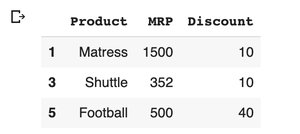Example 2 : if condition on row values (tuples) : This can be taken as a special case for the condition on column values. If a tuple is given (Sofa, 5000, 20) and finding it in the DataFrame can be done like :

 `# if condition with row tuple given``df[(df[``'Product'``] ``=``=` `'Sofa'``) & (df[``'MRP'``] ``=``=` `5000``) & (df[``'Discount'``]``=``=` `20``)]`

Output :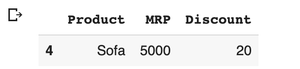Example 3 : Using Lambda function : Lambda function takes an input and returns a result based on a certain condition. It can be used to apply a certain function on each of the elements of a column in Pandas DataFrame. The below example uses the Lambda function to set an upper limit of 20 on the discount value i.e. if the value of discount > 20 in any cell it sets it to 20.

 `# importing pandas as pd ``import` `pandas as pd `` ` `# Create the dataframe ``df ``=` `pd.DataFrame({``    ``'Product'``: [``'Umbrella'``, ``'Matress'``, ``'Badminton'``, ``                ``'Shuttle'``, ``'Sofa'``, ``'Football'``],``    ``'MRP'``: [``1200``, ``1500``, ``1600``, ``352``, ``5000``, ``500``],``    ``'Discount'``: [``0``, ``10``, ``0``, ``10``, ``20``, ``40``]``})`` ` `# Print the dataframe ``print``(df) `` ` `# If condition on column values using Lambda function ``df[``'Discount'``] ``=` `df[``'Discount'``].``apply``(``lambda` `x : ``20` `if` `x > ``20` `else` `x)``print``(df)`

Output :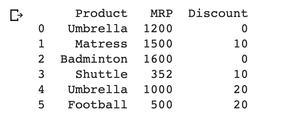Example 4 : Using `iloc()` or `loc() `function : Both `iloc()` and` loc() `function are used to extract the sub DataFrame from a DataFrame. The sub DataFrame can be anything spanning from a single cell to the whole table. `iloc()` is generally used when we know the index range for the row and column whereas `loc()` is used on a label search.

The below example shows the use of both of the functions for imparting conditions on the Dataframe. Here a cell with index [2, 1] is taken which is the Badminton product’s MRP.

 `# If condition on a cell value using iloc() or loc() functions``# iloc() is based on index search and loc() based on label search`` ` `# using iloc()``if` `df.iloc[``2``, ``1``] > ``1500``:``  ``print``(``"Badminton Price > 1500"``)``else``:``  ``print``(``"Badminton Price < 1500"``)`` ` `# using loc()``print``(df.loc[``2``, ``'MRP'``])``if` `df.iloc[``2``, ``'MRP'``] > ``1500``:         ``  ``print``(``"Badminton Price > 1500"``)``else``:``  ``print``(``"Badminton Price < 1500"``)`

Output :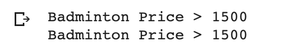My Personal Notes arrow_drop_up